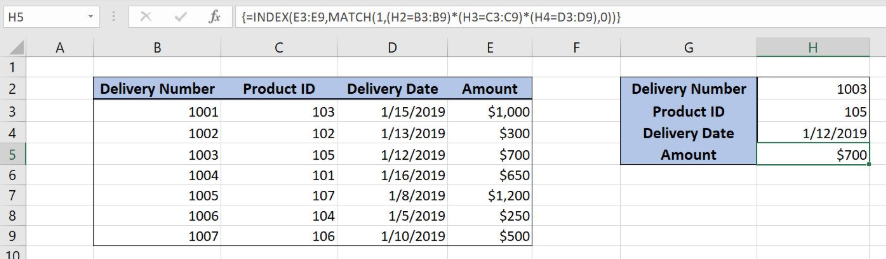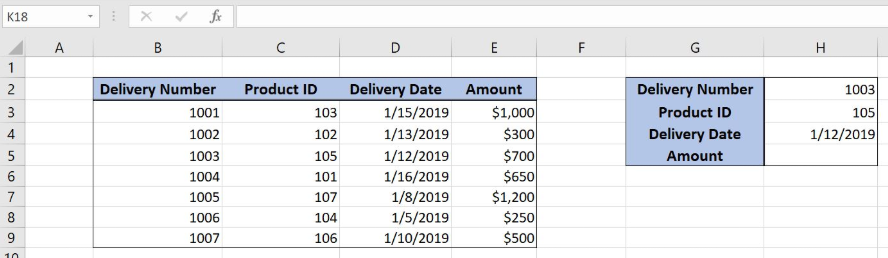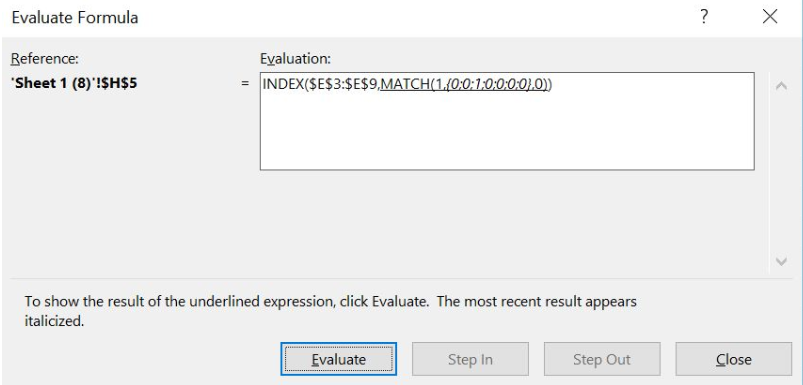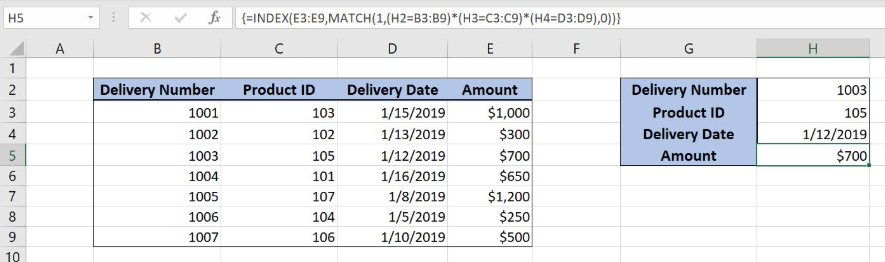Get instant live expert help with Excel or Google Sheets“My Excelchat expert helped me in less than 20 minutes, saving me what would have been 5 hours of work!”

#### Post your problem and you’ll get expert help in seconds.

Your message must be at least 40 characters
Our professional experts are available now. Your privacy is guaranteed.

# Tips on Performing an INDEX and MATCH with Two Criteria

Excel allows a user to do a lookup with two criteria using the INDEX and MATCH functions. The MATCH function returns a row for a value in a table, while the INDEX returns a value for that row. This step by step tutorial will assist all levels of Excel users in learning tips on performing an INDEX and MATCH with two criteria.Figure 1. The final result of the formula

## Syntax of the INDEX formula

The generic formula for the INDEX function is:

`=INDEX(array, row_num, column_num)`

The parameters of the INDEX function are:

• array – a range of cells where we want to get a data
• row_num – a number of a row in the array for which we want to get a value
• column_num – a column in the array which returns a value.

## Syntax of the MATCH formula

The generic formula for the MATCH function is:

`=MATCH(lookup_value, lookup_array, [match_type])`

The parameters of the MATCH function are:

• lookup_value – a value which we want to find in the lookup_array
• lookup_array – the array where we want to find a value
• [match_type] – a type of match. We put 0 as we want the exact match.

## Setting up Our Data for the Formula

Our table consists of 4 columns: “Delivery Number” (column B), “Product ID” (column C), “Delivery Date” (column D) and “Amount” (column E).  In the cell H5, we want to get the amount from the table, where the Delivery Number is equal to H2, the Product ID is equal to H3 and Delivery Date is equal to H4.Figure 2. Data that we will use in the example

## Performing an INDEX and MATCH with Two Criteria

We want to get an amount, from the lookup table B3:E9, where the Delivery number is 1003, the Product ID is 105 and the Delivery date is 1/12/2019.

The formula looks like:

`{=INDEX(E3:E9, MATCH(1, (H2=B3:B9) * (H3=C3:C9) * (H4=D3:D9), 0))}`

The lookup_value parameter of the MATCH function is 1. The lookup_array is `(H2=B3:B9) * (H3=C3:C9) * (H4=D3:D9)`. The cells H2, H3 and H4 are compared to the arrays and as a result arrays with 0 and 1 is returned. The match_type is 0, which means the approximate match. The result of the MATCH function is the row_num parameter of the INDEX function. The array is the range E3:E9.

To apply the formula, we need to follow these steps:

• Select cell H5 and click on it
• Insert the formula: `=INDEX(E3:E9,MATCH(1,(H2=B3:B9)*(H3=C3:C9)*(H4=D3:D9),0))`
• Press ctrl, shift and enter simultaneously to convert the formula in the array function.

When we evaluate all three comparations in the MATCH function, the lookup_array looks like in Figure 3.Figure 3. Evaluate the formula

Therefore, the MATCH function returns 3.Figure 4. Using the INDEX and MATCH Formula

The third row is the row_num parameter of the INDEX function. Finally, the amount from the third row of the lookup_table is the result of the function. The result in the cell H5 is \$700.

Most of the time, the problem you will need to solve will be more complex than a simple application of a formula or function. If you want to save hours of research and frustration, try our live Excelchat service! Our Excel Experts are available 24/7 to answer any Excel question you may have. We guarantee a connection within 30 seconds and a customized solution within 20 minutes.

Solution examplesindex and match with duplicates. I need to use another column as a reference, so my return value has two match the value of two things for it to return
Solved by E. H. in 60 minsHello, I have a big spreadsheet in which I need to know how many patients came in each month based on provider. I am using the following formula but I still get the N/A error. =INDEX('No Show Appts Data'!D:E, MATCH(1, 'No Show Appts Data'!D:D='No Shows Data'!M2)*('No Shows Data'!E:E='No Shows Data'!N1),0)) No Show Appts Data is the name of the sheet where Column D is Month Year of date in question and Column E is the provider. No Shows Data is the sheet where I am making all the formula calculation where Column M is Month and Year and Column N is the provider in question and therefore M2 is the month in question and N1 the provider in question. How do I fix this error? Thanks
Solved by F. H. in 40 minsI need to find an INDEX function that will convert the Call Day (a number 1-7) to the actual weekday found in row 1 of the DayofWeek named range. I don't know if I'm supposed to use the MATCH function as well or not.
Solved by D. D. in 11 minsI have two columns with names and a third one with email addresses. For every name that matches, I need to copy the email address to an empty column next to the matching name. .
Solved by E. U. in 15 minshelp with INDEX(IndexArea,MATCH(\$M\$5,MatchRows,0),MATCH(\$M\$6,MatchColumns,0))
Solved by Z. Y. in 12 mins